Courses
Courses for Kids
Free study material
Offline Centres
More

# Reciprocity TheoremLast updated date: 05th Dec 2023
Total views: 123.9k
Views today: 3.23k## An Introduction to Reciprocity Theorem

The reciprocity theorem is one of the most significant network theorems in electricity and currents. The theorem was introduced by Max Theodor Felix von Laue. According to the reciprocity theorem, the value of current obtained in any branch of an electrical circuit or network due to a single voltage source (V) is equal to the value of current flowing through that branch when the source was first connected and when it was connected to the branch where the value of current was initially determined. The reciprocity theorem is described along with its statement and proof in the article.

## History of Max Theodor Felix Von Laue

Max Theodor Felix von Laue, a German scientist who lived from 9 October 1879 to 24 April 1960, won the Nobel Prize in Physics in 1914 for his invention of the diffraction of X-rays by crystals. In 1935, von Laue demonstrated how to apply the reciprocity theorem to simplify the issue.Max Theodor Felix Von Laue

## Reciprocity Theorem Statement

Reciprocity Theorem states that the output reading of the ammeter in any electrical circuit would remain unchanged if the voltage source and ammeter were switched places.

In simple words, the reciprocity theorem implies that no matter how the current and voltage sources are arranged in a network, the magnitude of the current and voltage that flows through the circuit remains constant.

## Steps for Using the Reciprocity Theorem to Solve a Network

• Decide which branches need reciprocity to be developed first.

• Consider that the sources are located in the branch x-y.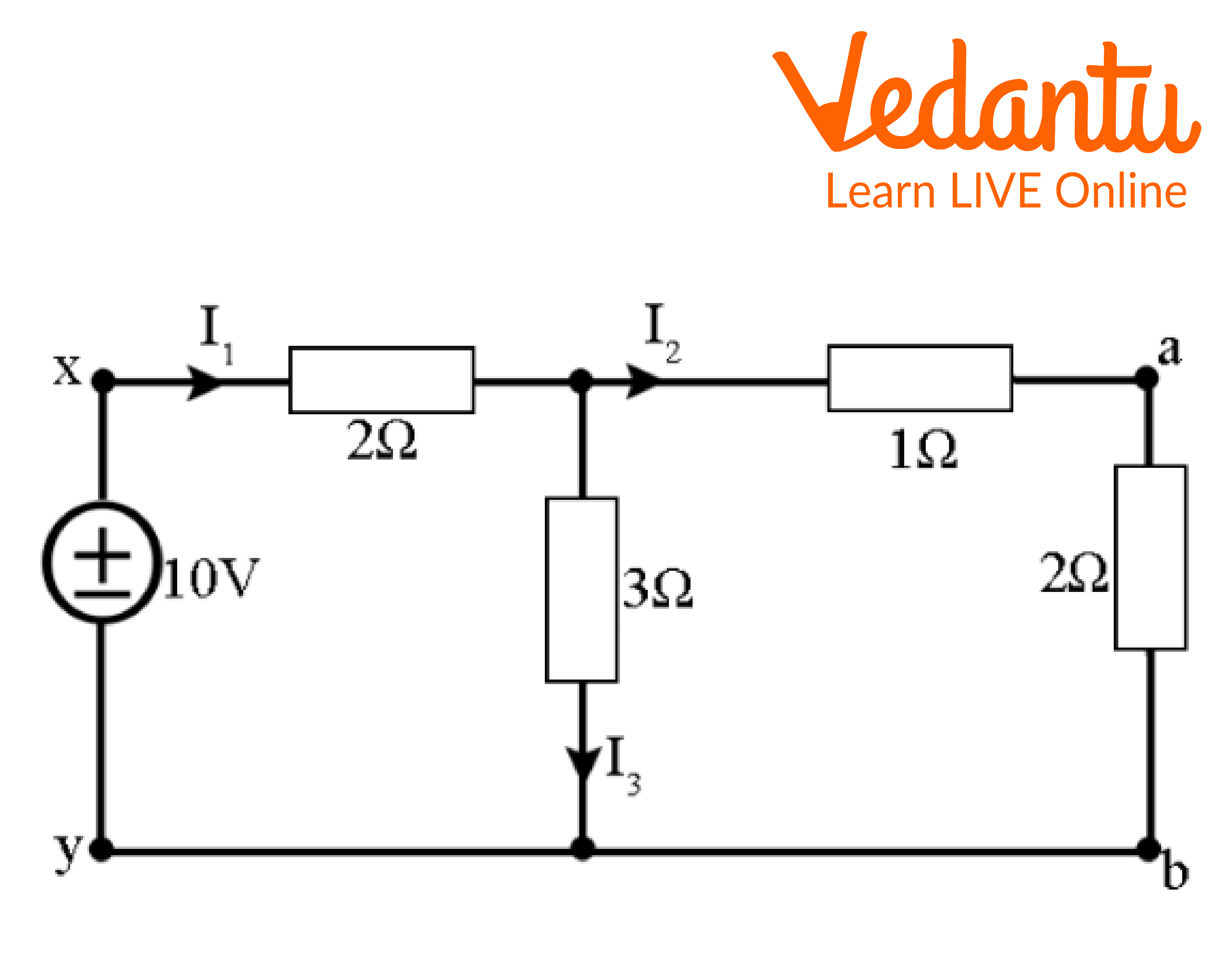Reciprocity Theorem

• The corresponding resistance across x-y, with reference to above figure, is given by

${R_{eq\;}}\; = \;\left[ {\left( {2 + 3} \right)||3} \right]\; + 2\;\\{R_{eq\;}}\; = \;3.5\Omega \\\therefore \;{I_1}\; = \dfrac{{\;10}}{{3.5}}\; = \;2.86A\\ \Rightarrow {I_2}\; = 2.86 \times \dfrac{3}{{3 + 3}}\; = \;1.43A\\ \Rightarrow {I_3}\; = \left( {2.86\; - 1.43} \right)\; = \;1.43A$

• Any typical network analysis technique can be used to determine the current in the branch.

• The branch that is chosen switches out the voltage source.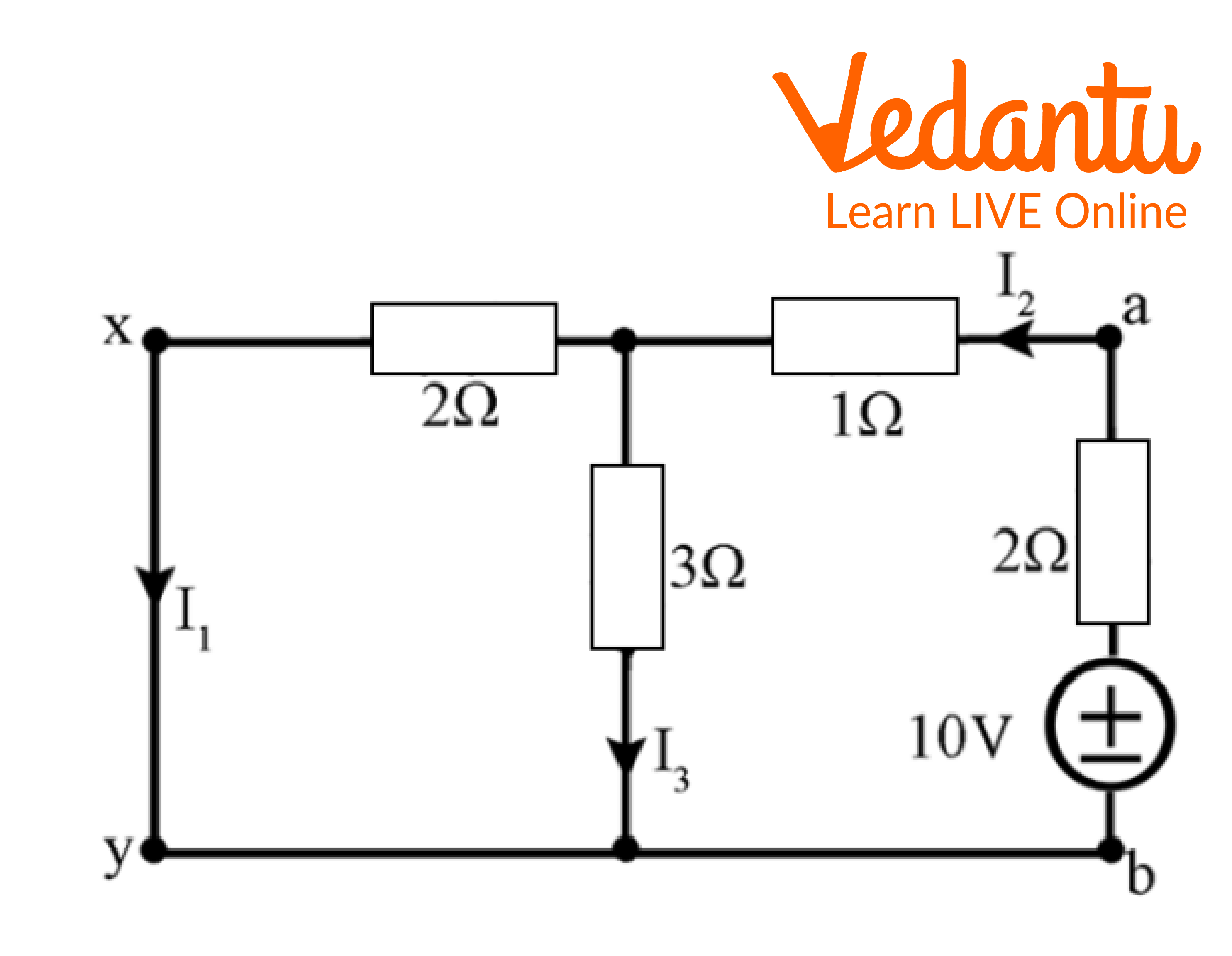Reciprocity Theorem

${R_{eq\;}}\; = \;\left( {2||3} \right) + 1 + 2\;\;\\{R_{eq\;}}\; = \dfrac{6}{5} + 3\; = \;4.2\Omega$

$\therefore {I_{2\;}}\; = \dfrac{{\;10V}}{{4.2V}}\; = \;2.381$

$\Rightarrow {I_1}\; = \;2.381 \times \dfrac{3}{{3 + 2}}$

$\Rightarrow {I_1}\;\; = \;1.43A$

• The branch where the previous voltage source was located has its current calculated.

• Now it can be observed that the current measured in the previous connection, or step 1, and the current computed when the source is switched, or in step 3, are the same.

## Limitations of Reciprocity Theorem

• Not applicable to circuits with any type of time-varying component.

• Not relevant to circuits with dependent sources, even if they are linear.

• Not applicable to circuits made up of nonlinear components like transistors, diodes, etc.

## Application of Reciprocity Theorem

• The reciprocity theorem can be applied to circuits with either a current source or a voltage.

• This theorem is used to examine the ultrasonic produced when elastic bodies are heated to high temperatures on their surfaces.

• This theorem is used to calculate surface waves caused by line loads on an irregular, transversely isotropic half-space.

## Solved Examples

1. Does the reciprocity theorem apply to any of the following networks?

1. Containing both dependent and independent sources.

2. Having R, L, and C elements.

3. A system that is initially not relaxed.

Ans: Yes, the reciprocity theorem applies to the networks containing R, L, and C elements. So, the only viable option is option B because options A and C don't satisfy the requirements for applying this theorem.

2. What will be the value of being ${I_2}$ in the following figure if ${I_1}$in the given circuit is 6A?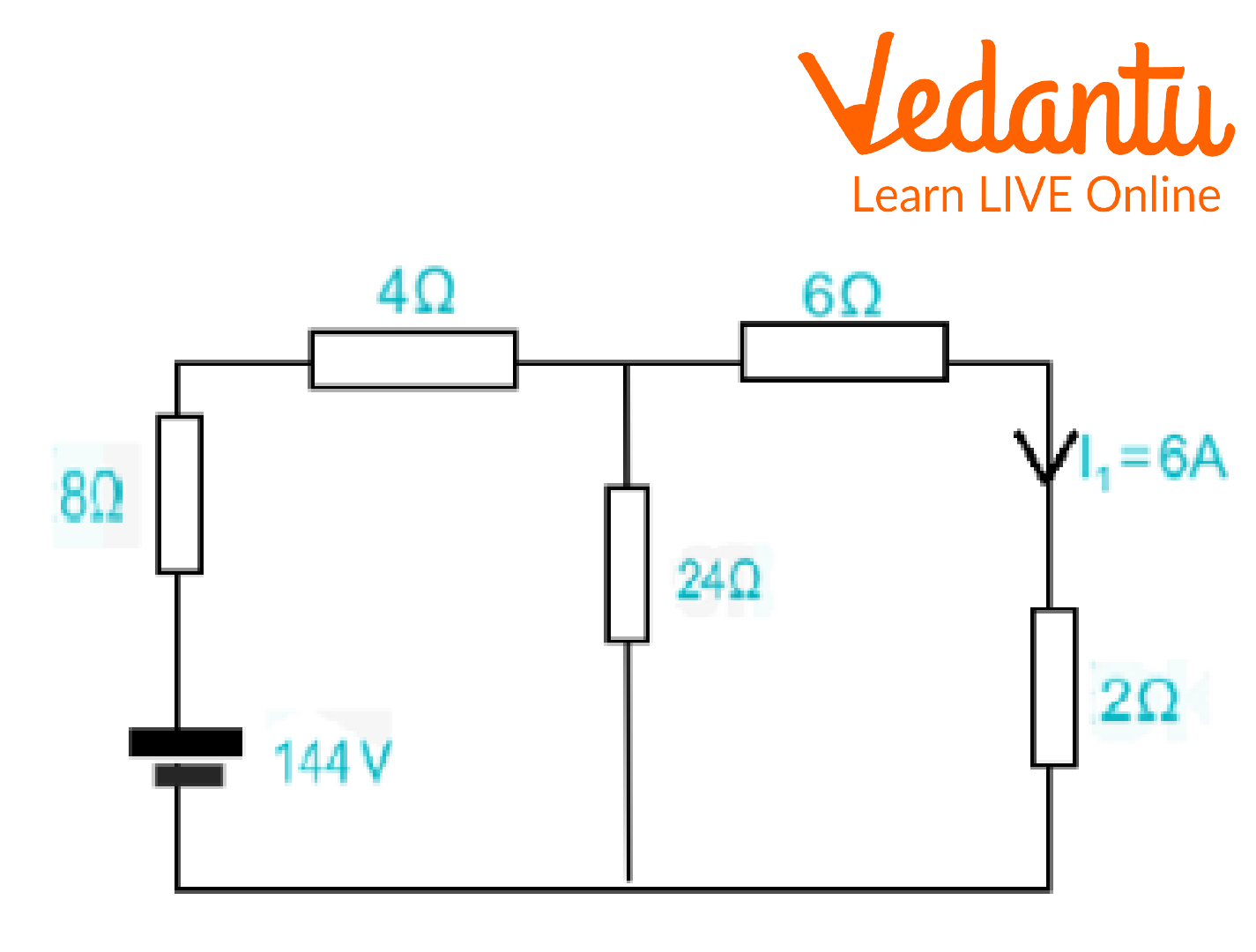Circuit Diagram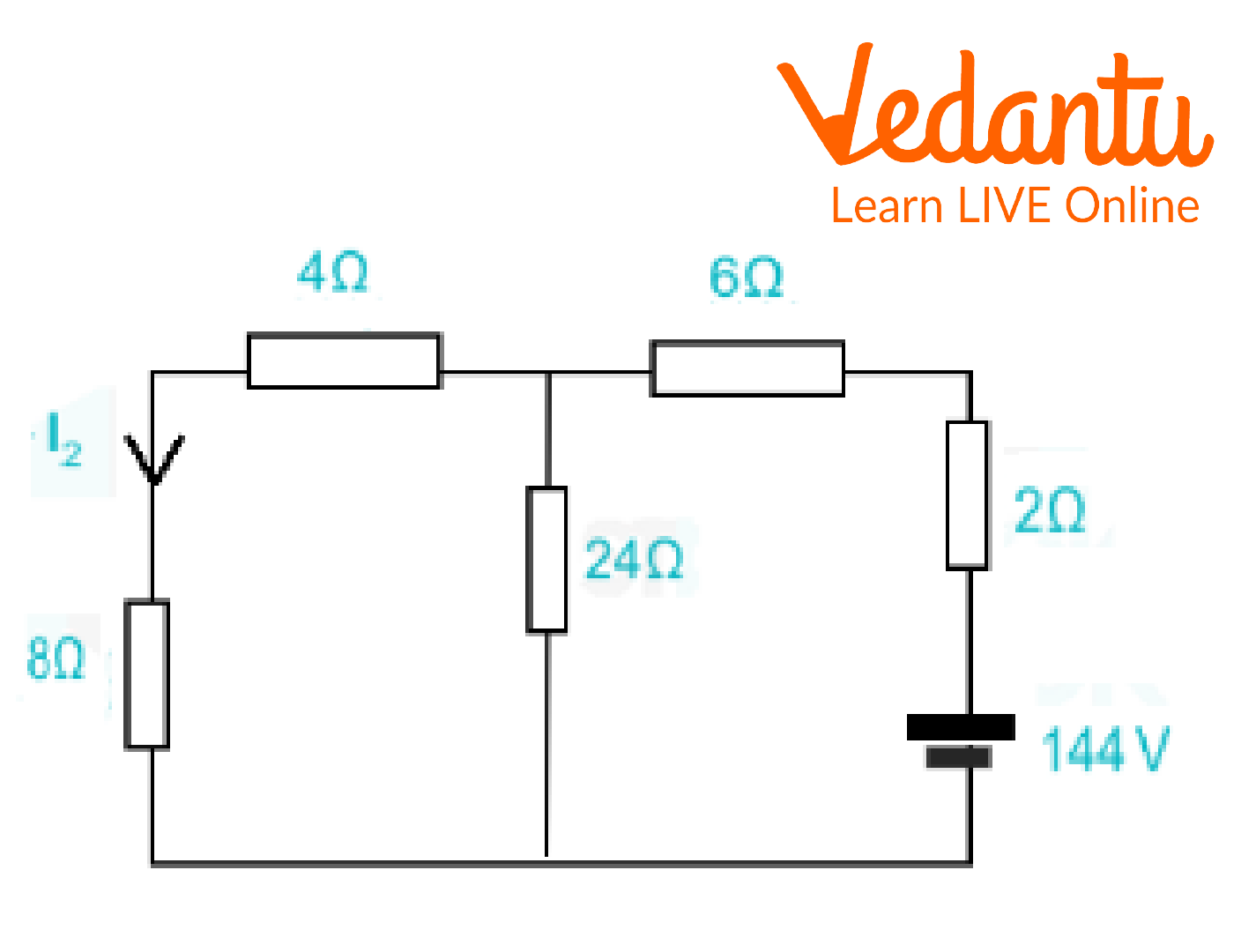Circuit Diagram

Ans: Given data,

${I_1}\; = \;6V$

${V_{1\;}}\; = \;144V$

${V_2}\; = \;\;144V$

${I_2}\; = \;?$

If a single voltage source v in the branch AB of any passive bilateral network results in a current response I in the branch CD, then the voltage source's removal from the branch AB and its insertion into the branch CD will result in the same current in the branch AB.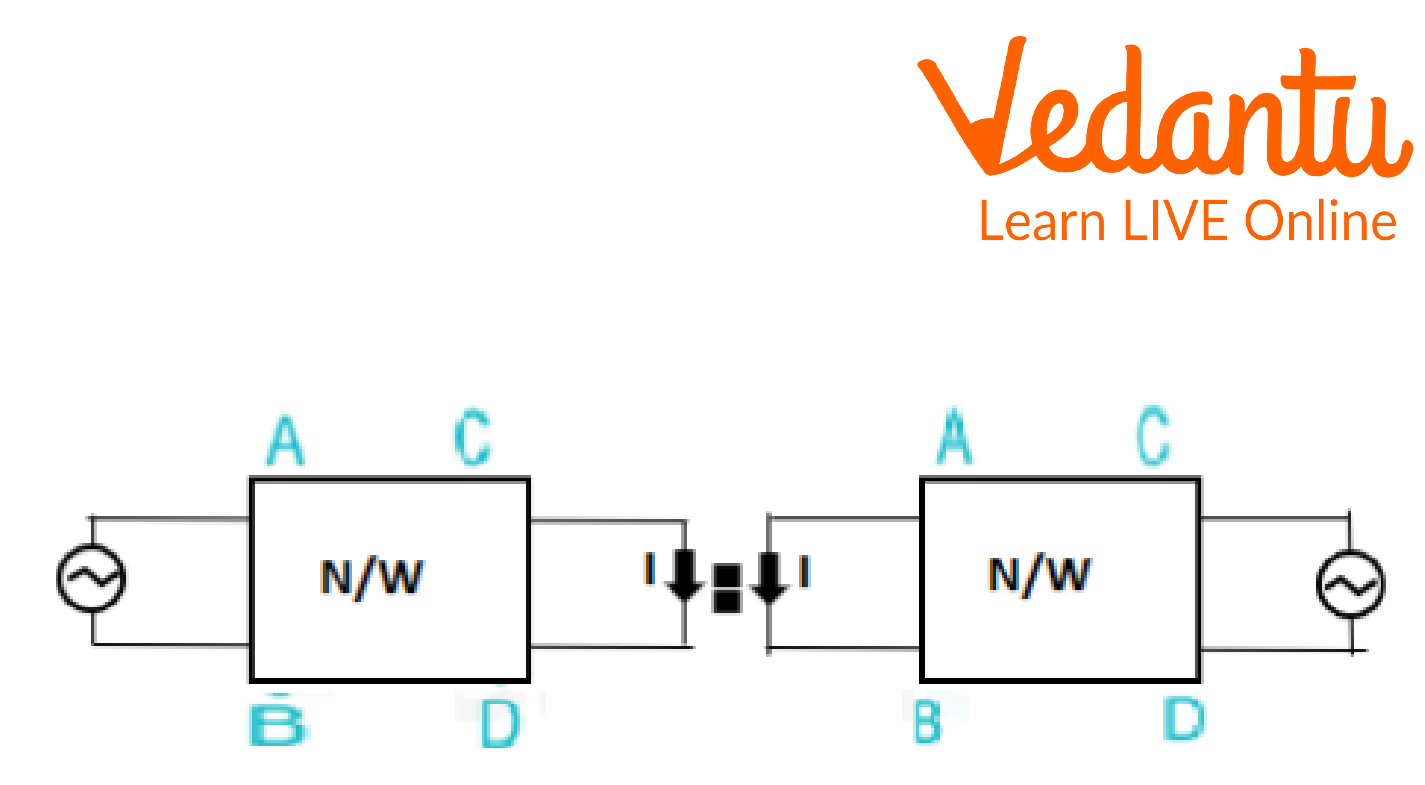Circuit Diagram of Reciprocity Theorem

If the positions of excitation and responses are switched in the reciprocity theorem, the ratio stays the same.

$\dfrac{{{V_1}}}{{{I_1}}} = \dfrac{{{V_2}}}{{{I_2}}}$

Replace the terms with the known values,

$\dfrac{{144}}{6}\; = \dfrac{{\;144}}{{{I_2}}}$

$144{I_2}\; = \;864\\{I_2}\; = \dfrac{{\;864}}{{144}}$

${I_{2\;}}\; = \;6\;A$

3. Demonstrate how the reciprocity theorem is applied to the network in the accompanying diagram.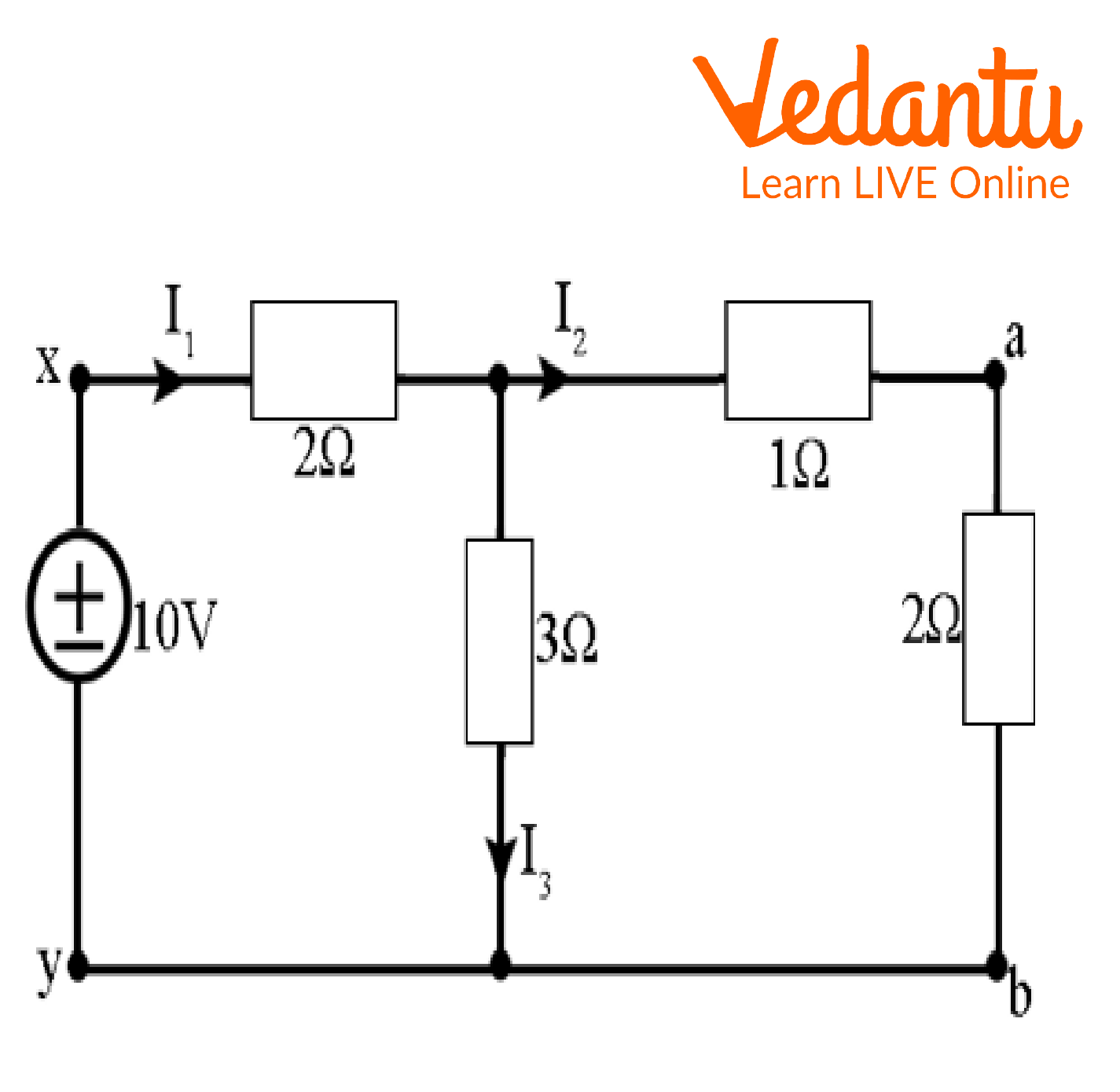Circuit Diagram

Ans: The comparable resistance across x-y concerning the following figure is given by,Circuit Diagram

${R_{eq}}\; = \;\left[ {\left( {2 + 1} \right)||3} \right] + 2\;$

${R_{eq}}\; = \;3.5\Omega$

$\therefore {I_1}\; = \dfrac{{\;10}}{{3.5}}$

${I_1}\; = \; = \;2.86A$

${I_2}\; = \;2.86 \times \dfrac{3}{{3 + 3}}$

I2 = 1.43A

${I_3}\; = \;2.86 - \;1.43$

${I_3}\; = \;1.43A$

Now,Circuit Diagram

${R_{eq}}\; = \;\left( {2||3} \right) + 1 + 3$

${R_{eq}}\; = \dfrac{{\;6}}{5} + 3$

${R_{eq}}\; = \dfrac{{\;21}}{5}$

${R_{eq}}\; = 4.2\Omega$

$\therefore {I_2}\; = \dfrac{{\;10V}}{{4.2\Omega }}$

${I_2}\; = \;2.381A$

This implies

${I_1}\; = \;{I_2}\dfrac{3}{{3 + 2}}$

${I_1}\; = \;2.381 \times \dfrac{3}{5}$

${I_1}\; = \;1.43A$

### Important Points to Remember

• Both AC and DC circuits are covered by the reciprocity theorem.

• The circuit does not contain any time-varying components when applying the theorem.

• It is feasible to determine if a network is linear or non-linear using the reciprocity theorem.

## Conclusion

One of the most crucial electromagnetics theorems is the reciprocity theorem. This fundamental theorem explains that even if the locations of a network's voltage and current sources are switched, the circuit's current and voltage levels will remain constant. Many DC and AC networks that have numerous uses in electromagnetic electronics are solved using this theorem. There isn't a time-varying component in these circuits.

## FAQs on Reciprocity Theorem

1. What is the antenna's use of the reciprocity theorem?

Reciprocity, which states that the characteristics of transmitting and an antenna are equivalent, is the attribute of an antenna that is most helpful. As a result, antennas do not have distinct transmit and receive radiation patterns. If we are familiar with the radiation pattern in the broadcast mode, we can identify the pattern in the receive mode.

An antenna's primary purposes are for receiving and sending signals. Once the working mode of the antenna has been altered, characteristics can also be altered. The reciprocity property refers to the fact that the antenna's attributes remain unaltered.

2. What is Lorentz's Reciprocity Theorem?

One of the most prevalent and general theorems is the Lorentz's reciprocity theorem, which includes several particular examples like Rayleigh-Carson reciprocity. Following similar conclusions for sound by Lord Rayleigh and light by Helmholtz (Potton, 2004), it was given the name Hendrik Lorentz in 1896. Most of the electrical and photonic structures share the fundamental property of Lorentz reciprocity. The Lorentz reciprocity theorem in electromagnetic field theory describes the energy coupling between fields generated by one source on another and vice versa.

3. Is the circuit used to apply the reciprocity theorem simple or complex?

The reciprocity theorem-applied circuit could be simple or complex. Each intricate reciprocal network, however, can be reduced to a straightforward circuit. Based on the reciprocity theorem, both the voltage supply and the output current are movable equally in a linear passive network. So, the theorem can be applied to circuits with either a current source or a voltage.

But the theorem does not apply to the circuits containing any time-varying components. Otherwise, it is feasible to determine if a network is linear or non-linear using the reciprocity theorem.